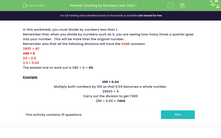# Divide by Numbers Less Than 1

In this worksheet, students will practise decimal calculations by dividing by numbers less than 1.Key stage:  KS 3

Curriculum topic:   Number

Curriculum subtopic:   Use Four Operations for All Numbers

Difficulty level:#### Worksheet Overview

In this activity, we will divide by numbers less than 1.

Remember that, when you divide by numbers such as ¼, you are seeing how many times a quarter goes into your number.  This will be more than the original number - don't worry - it's meant to be!!Remember also that all the following divisions will have the SAME answers:

2400 ÷ 40

240 ÷ 4

24 ÷ 0.4

2.4 ÷ 0.04

The easiest one to work out is 240 ÷ 4 = 60.

Example

296 ÷ 0.04

The first thing to do is make the number you're dividing by into a whole number. In this case, we need to multiply 0.04 by 100. Remember that whatever we do to that number, we have to do the same thing to the number you're dividing!

So, we need to multiply both numbers by 100 to make 0.04 become a whole number.

297 x 100 = 29,600

0.04 x 100 = 4

This gives us 29,600 ÷ 4 which is much easier!

Carry out the division to get 7,400

296 ÷ 0.04 = 7,400

Let's have a go at some questions now.

### What is EdPlace?

We're your National Curriculum aligned online education content provider helping each child succeed in English, maths and science from year 1 to GCSE. With an EdPlace account you’ll be able to track and measure progress, helping each child achieve their best. We build confidence and attainment by personalising each child’s learning at a level that suits them.

Get started